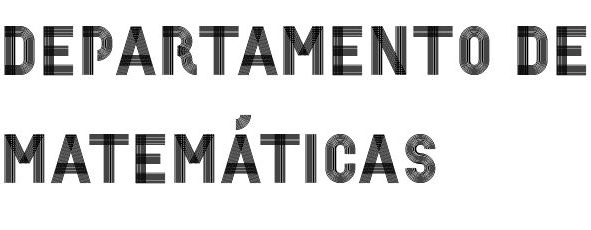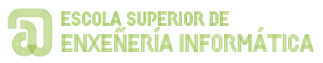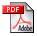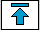HOME PROCEEDINGS OTHER PAPERS

 An abstract averaging method with applications to differential equations, J. Differential Equations  274, 15, (2021), 231-250 (with J. Mawhin and M. Zima).On the existence of periodic oscillations for pendulum-type equations, Adv. Nonlinear Anal. 2021; 10: 121-130.Nonnegative oscillations for a class of differential equations without uniqueness: a variational approach, 25 (2) (2020), 545-554 (with L. Sánchez).Lyapunov stability of periodic solutions of Brillouin type equations, 101 (2020), 106057, 7 pp. (with F. Wang, S. Li and M. Zima).Lyapunov stability for regular equations and applications to the Liebau phenomenon, Discrete Contin. Dyn. Syst. 38, 9, (2018), 4657–4674 (with F. Wang and M. Zima).A Lipschitz condition along a transversal foliation implies local uniqueness for ODEs, Electronic Journal of Qualitative Theory of Differential Equations 2018, No. 13, 1–14 (with F. A. F. Tojo).A non-variational approach to the existence of nonzero positive solutions for elliptic systems,  J. Fixed Point Theory Appl. 19 (2017), 3151-3162 (with G. Infante).New results for the Liebau phenomenon via fixed point index, Nonlinear Analysis Series B: Real World Applications 35 (2017) 457-469 (with G. Infante, M. Tvrdy and M. Zima).Green's Functions and Spectral Theory for the Hill's Equation, Appl. Math. Comput. 286 (2016) 88-105 (with A. Cabada and L. López-Somoza).Integration by parts and by substitution unified with applications to Green's Theorem and uniqueness for ODEs, Amer. Math. Monthly 123 (2016) 40-52 (with R. L. Pouso). See an added endnote.A generalization of Montel–Tonelli's Uniqueness Theorem, J. Math. Anal. Appl. 429 (2015) 1173-1177 (with R. L. Pouso).A topological approach to periodic oscillations related to the Liebau phenomenon, J. Math. Anal. Appl. 423 (2015) 1546-1556. (with G. Infante, M. Tvrdy and M. Zima).A positive fixed point theorem with applications to systems of Hammerstein integral equations, Bound. Val. Probl. (2014) 2014 :254 (with A. Cabada and G. Infante).Heteroclinics for some non autonomous third order differential equations, Topol. Methods Nonlinear Anal. 43  (2014) 53-68 (with D. Bonheure, C. De Coster and L. Sanchez).On the pumping effect in a pipe/tank flow configuration with friction, Physica D  273-274 (2014) 28-33 (with G. Propst and M. Tvrdý).New criteria for the existence of non-trivial fixed points in cones, to appear in Fixed Point Theory and Applications, 2013:125, doi: 10.1186/1687-1812-2013-125 (with A. Cabada and G. Infante).On the existence and stability of periodic solutions for pendulum-like equations with friction and $\phi$-Laplacian,  Discrete Contin. Dyn. Syst. 33 1 (2013), 141-152 (special issue: tribute to J. Mawhin) (with Pedro J. Torres).On comparison principles for the periodic Hill's equation, J. Lond. Math. Soc. 86 (2012)  272-290, doi: 10.1112/jlms/jds001 (with A. Cabada).Existence of infinitely many solutions for second-order singular initial value problems with an application to nonlinear massive gravity, Nonlinear Analysis Series B: Real World Applications 12 (2011) 2596-2606, doi:10.1016/j.nonrwa.2010.09.030 (with O. López-Pouso and R. López-Pouso).Existence and multiplicity of solutions for a periodic Hill's equation with parametric dependence and singularities, Abstract and Applied Analysis Volume 2011 (2011), Article ID 545264, 19 pages, doi:10.1155/2011/545264 (with A. Cabada).On a class of singular Sturm-Liouville periodic boundary value problems, Nonlinear Analysis Series B: Real World Applications 12 (2011) 2378-2384, doi:10.1016/ j.non rwa.2011.02.010 (with A. Cabada).Existence of solutions for elliptic systems with nonlocal terms in one dimension, Boundary Value Problems, vol. 2011, Article ID 518431, 12 pages, 2011, doi:10.1155 /2011/518431. Special issue on "Nonlocal Boundary Value Problems" (with A. Cabada and L. Sanchez).A generalized anti-maximum principle for the periodic one dimensional $p$-Laplacian with sign changing potential, Nonlinear Anal. 72 (2010) 3436-3446; doi:10.1016 / j.na.2009.12.028 (with A. Cabada and M. Tvrdý).Solvability of some $\phi$-Laplacian singular difference equations defined on the integers, AJSE - Mathematics 1, no. 1, (2009) 75-81. (with A. Cabada).Positive fixed points and fourth-order equations, Bull. Lond. Math. Soc. 41 (2009), 72-78, doi:10.1112/blms/bdn105 (with D. Franco and F. Minhós).Existence of a non-zero fixed point for nondecreasing operators via Krasnoselskii's fixed point theorem, Nonlinear Anal. 71 (2009), 2114-2118; doi: 10.1016/j.na.2009.01.045, (with A. Cabada).Solvability for some boundary value problems with $\phi$-Laplacian operators, Discrete Contin. Dyn. Syst. 23 (2009), 727-732, (with P. J. Torres).Existence of unique solutions for perturbed autonomous differential equations, J. Lond. Math. Soc. 78 (2008) 798-812; doi: 10.1112/jlms/jdn058 (with R. L. Pouso).On the sign of the Green's function associated to Hill's equation with an indefinite potential, Appl. Math. Comput. 205 (2008), 303-308, (with A. Cabada).Existence of solution for a singular differential equation with nonlinear functional boundary conditions, Glasg. Math. J. 49 (2007), 213-224, (with A. Cabada).Sharp conditions for the existence of solutions for second-order autonomous differential equations, Mediterr. J. Math. 4 (2007), 191-214,  (with R. L. Pouso  and R. Roque Enguiça).Initial value problems for singular and nonsmooth second order differential inclusions, Math. Nachr. 280  (2007), 1335-1343,  (with D. C. Biles and R. L. Pouso).Positivity and lower and upper solutions for fourth order boundary value problems, Nonlinear Anal. 67  (2007), 1599-1612,  (with A. Cabada and L. Sanchez).Solvability of discontinuous functional differential systems in $l_{\infty}(M)$,  ANZIAM J. 48 (2006), 237-243,  (with A. Cabada and S. Heikkilä).Uniqueness and existence results for ordinary differential equations, J. Math. Anal. Appl. 316 (2006), 178-188, (with S. Heikkilä and R. L. Pouso).On extending existence theory from scalar ordinary differential equations to infinite quasimonotone systems of functional equations,  Proc. Amer. Math. Soc. 133 (2005),  2661-2670.Ordinary differential equations and systems with time-dependent discontinuity sets, Proc. Roy. Soc. Edinburgh Sect. A 134 (2004), 617-637, (with R. L. Pouso).The uniqueness of fixed points for decreasing operators, Appl. Math. Lett., 17 (2004), 861--866.A note on fixed point theorems for T-monotone operators, Comput. Math. Appl., 47 (2004),  853- 857, (with A. Cabada).On extremal fixed points in Schauder's theorem with applications to differential equations,  Bull. Belg. Math. Soc. Simon Stevin, 11 (2004), 15--20.Periodic solutions for second order differential equations with discontinuous restoring forces, J. Math. Anal. Appl., 288 (2003), 349--364, (with L. Sanchez).On uniqueness criteria for systems of ordinary differential equations, J. Math. Anal. Appl., 281 (2003), 264--275.On first order ordinary differential equations with non-negative right-hand sides, Nonlinear Anal., 52 (2003),  1961--1977, (with R. L. Pouso).Última actualización: